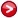# Full list of tree diagrams implementing the d=9,11,13 neutrino mass operators

In the paper "Gaetana Anamiati, O. Castillo-Felisola, Renato M. Fonseca, Juan C. Helo and Martin Hirsch, High-dimensional neutrino masses, arXiv:1806.07264 [hep-ph]"we considered all possible tree-level realizations of the neutrino mass operators $LLHH(HH^*)^{(d-5)/2}$ with $d=9,11,13$.

The complete list of diagrams, as pdf files, can be found here: $d=9$, $d=11$and $d=13$.

For automated processing of theses lists of diagrams, we also provide herethe same data as a Mathematica file (plus an auxiliar file).Author
Renato Fonseca

Emails
renatofonseca@gmail.com
or
renatofonseca@ugr.es

Last updated
13 December 2021